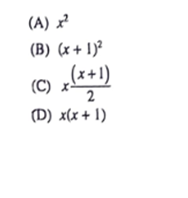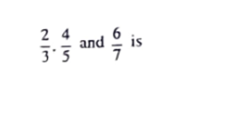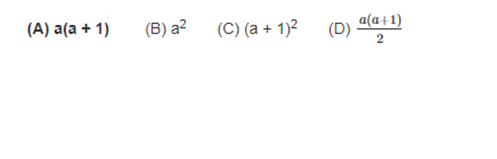## WBCS MAIN PAPER VI : MATHEMATICS - LCM AND HCF

1.If x is a prime number, the LCM of x and (x + 1) is2.HCF of the fractions(A) 481105

(B) 2/105

(C) 24/105

(D) 1/105

3.The HCF of two numbers is 4 and LCM is 520. If one of the numbers is 52 the other number is then

(A) 40

(8) 42

(C) 52

(D) 50

4.If the ratio of three numbers are 3:4:5 and their LCM is 1200, then the smallest number is

(A) 80

(B) 60

(C) 120

(D) 100

1.The ratio of two numbers is 3 : 4 and their L.C.M. is 180. Find the numbers.

(A) 45, 60        (B) 40, 25        (C) 50, 30        (D) 30, 60

1.The product of two numbers is 1320 and their H.C.F. is 6. The L.C.M. of number is

(A) 220            (B) 1314          (C) 1326          (D) 7920

1.If a is a prime number, then the L.C.M. of a and (a + 1) is2.The H.C.F. and L.C.M. of two numbers x and y are respectively 3 and 105. If x+ y = 36, then  1/x  + 1/y = ?

(A) 4/36 (B) 1/35 (C) 35       (D) 3 /5

3.The H.C.F. of two numbers is 12 and their difference is also 12. The numbers are

(A) 66, 76        (B) 70, 82        (C) 94, 108        (D) 84, 96

4.The H.C.F. of 0.54, 1.8 and 7.2 is

(A) 0.18     (B) 1.8             (C) 0.018         (D) 18

1.If the ratio of three numbers is 3 : 4 : 5 and their LCM is 1200, then the smaller number is :

(A) 60      (B) 80              (C) 100        (D) 120

2.The product of two co-primes is 117 ; their L.C.M. should be :

(A) 1   (B) 117        (C) equal to their H.C.F.             (D) Cannot be calculated

1.The H.C.F. of three numbers is 12. If they are in the ratio 1 : 2 : 3, then the numbers are

(A) 6, 12, 18   (B) 10, 20, 30       (C) 12, 24, 36          (D) 24, 48, 72

2.If two numbers are in the ratio 5 : 7 and their L.C.M. is 315, then their product is

(A) 2358          (B) 2385          (C) 2538       (D) 2835

3.The product of two Co-primes is 117. Their L.C.M. should be

(A) 1        (B) 117         (C) equal to their H.C.F.     (D) Cannot be calculated

1. The H.C.F. and L.C.M. of three numbers are 6 and 420.  If two numbers be 12 and 30, the third number will be -

(A) 21  (B) 18       (C) 42          (D) 70

2.The HCF of two numbers is 145 and their LCM is 2175. If one of the number is 725, the other number is-

(A) 290        (B) 435    (C) 5   (D) none of the above

Shopping Cart